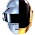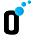## Factorial Trailing Zeroes

Given an integer n, return the number of trailing zeroes in n!.
Note: Your solution should be in logarithmic time complexity.

### Analysis:

Zeros can only generated on prime factors of 5s and 2s.
Lets' say 5s = {5*1, 5*2 ... 5*n}, 2s = {2*1, 2*2 ... 2*n}

So in this problem we need to count how many 5s and 2s.
Note that in n!, the 2s are always more than 5s, so we simplify the problem into counting the 5s.
There are several cases on 5s.  They are 5, 5^2, 5^3 ...
First let's see how to count 5s,  all we need is to compute floor (n/5) .
Then let's deal with 5^n.
E.g., n = 28,  it has 5s =  [5, 10, 15, 20, 25]
When we count 5s using floor(n/5), we have the length 5, but 25 = 5*5, there should be another 5 and the length is 6.  To count this, we can continue counting 5s using n divide by 5, 5^2, 5^3 ... In this way, all the 5s can be found.

### Code(C++):

class Solution {
public:
int trailingZeroes(int n) {
long p = 5;
int res = 0;
while (p <= n){
res += n/p;
p = p*5;
}
return res;
}
};


### Code(Python):

class Solution(object):
def trailingZeroes(self, n):
"""
:type n: int
:rtype: int
"""
p = 5
res = 0
while p <= n :
res += n/p
p = p *5
return res



1.Elegant solution.

2.Very nice blog...... Hot Tot Hair Products seems to be very useful.... I would like to try them if i could buy them in india.... Nice work, keep it up.

Best iPhone 5 Cases

3.Good Code! If you are looking for iPhone 5 Cases in India then have a look on our site!